# A New-Type Semi-Rigid Base Layer Structure for Long Service Life Pavement

A New-Type Semi-Rigid Base Layer Structure for Long Service Life Pavement
College of Construction Engineering, Jilin University, Changchun 130026, China

Corresponding Author Email:
tianbw19@jlu.edu.cn
Page:
140-145
|
DOI:
https://doi.org/10.18280/eesrj.080305
12 June 2021
|
Accepted:
23 August 2021
|
Published:
30 September 2021
| Citation

OPEN ACCESS

Abstract:

Targeting at the problem of pavement cracking under long-term load, this study developed a new-type semi-rigid base layer structure based on the CGC (cement stabilized macadam - graded broken stone - cement stabilized macadam) combinations, and used ANSYS to simulate this proposed structure under conditions of different modulus combinations, deflection under different thickness, different vertical strain values on the top surface of roadbed, and different transverse tensile stress values of bottom base layer. The simulation results indicate that, the various mechanical properties of the proposed new structure can well meet the specifications, and the time of crack generation has been slowed down; the use of graded broken stone in the proposed structure has achieved both the purposes of saving construction cost and reducing construction difficulty. By reasonably controlling the CGC structure and modulus, this study has successfully suppressed the generation of reflection cracks, which can provide good theoretical evidence for prolonging the service life of semi-rigid base layer pavement.

Keywords:

asphalt pavement, finite element, reflection cracks, stress distribution, road performance

1. Introduction

2. Pavement Modeling

2.1 Basic assumptions 

Following assumptions are usually made for elastic layered systems:

1. The materials of each layer of the pavement are uniform and isotropic;
2. The soil foundation is a semi-infinite three-dimensional body which extends infinitely in the horizontal plane but is limited in the depth direction.
3. When horizontal and vertical loads are acting on the pavement surface, it is assumed that the stress and deformation at infinity (depth and horizontal directions) are zero;
4. Three assumptions for the contact surface between layers: the surface is completely continuous, completely smooth, and there’re relative displacements between layers.

2.2 Structure of asphalt pavement

In ANSYS simulation, the influence of boundary size had been minimized as much as possible . Therefore, the constructed semi-rigid asphalt base layer pavement model had a size of 10m×xm×10m, (length, height, and width), the pavement model was divided into 7 layers, from top to bottom, they are: top surface layer, middle surface layer, lower surface layer, top base layer, middle base layer, lower base layer, and soil base layer. Solid185 units were used to build the model, and there’re a total of 81608 units in the model, as shown in Figure 1.

## 1.jpg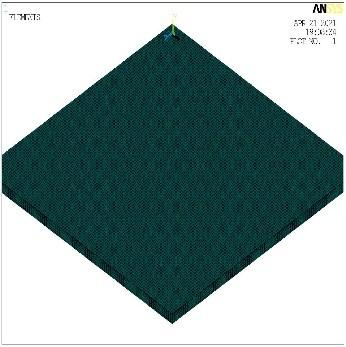Figure 1. Model of the pavement

2.3 Selection of material parameters

In terms of material selection, all layers in the model were elastic layered structures, the material parameters of each structure layer are listed in Table 1 below.

Table 1. Structure layers and material parameters of the pavement model 

 Structure layer Thickness/cm Elastic modulus/Mpa Poisson’s ratio Asphalt top surface layer AC-13 5 1200 0.25 Asphalt middle surface layerAC-16 5 1450 0.25 Asphalt lower surface layerAC-20 10 1950 0.25 Cement stabilized macadam 10/5/10/5 800/900/1000/1100/1200 0.4 Graded broken stone 10/20/20/30 300/340/380/420/460 0.3 Cement stabilized macadam 10/5/10/5 800/900/1000/1100/1200 0.4 Soil base - 60 0.35

The standard axle load of the pavement was indicated by BZZ-100, the value of axle load took 100KN, and the wheel pressure was 0.7Mpa [9, 10]. In this paper, based on the equivalent tire contact area, the shape of the contact area between the wheel and the ground was simplified into a rectangle with a length of 0.21m and a width of 0.167m, the distance between the centers of two wheels was 1.3m, see Figure 2.

## 2.png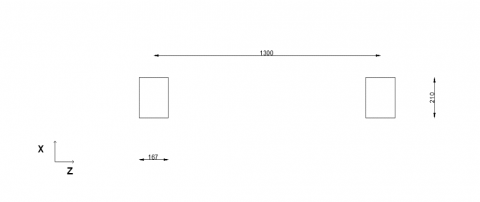Figure 2. Shape of the contact area between wheel and ground

2.5 Boundary conditions

To simulate real pavement conditions, it’s necessary to set boundary conditions for the pavement model. In the Y direction, namely the structure depth direction, the bottom position was fixed; there’s no displacement on the left and right sides of X direction, namely the driving direction; and there’s no Z-direction displacement on both sides of the cross section.

3. Calculation of Standard Test Pavement Structure

3.1 Vertical strain of the top surface of roadbed

The allowable vertical compressive strain on the top surface of roadbed was calculated by Formula 1.

[εz]=1.25×104-0.1β (kT3Ne4)-0.21     (1)

where,

[εz] is the allowable vertical compressive strain on the top surface of roadbed (10-6);

β is the reliability index, according to the highway grade, its value took 1.28 in this paper;

Ne4 is the cumulative number of equivalent design axle loads on the designed lane within the designed service life, its value was 12000000;

kT3 is the temperature adjustment coefficient, its value took 1.129;

After calculation, it’s obtained that εz =0.29595×10-3.

3.2 Transverse tensile stress of the bottom base layer

Table 2. Allowable tensile stress of structure layers

 Structure layer Allowable tensile stress/Mpa Asphalt pavement layer 0.465 Base layer 0.323 Bottom base layer 0.087

The HPDS pavement design software allows users to design highways of different grades according to the latest highway design specifications, the purpose of this software is to alleviate the workload of constructors, reduce errors in manual calculations, and make the various grades of highways be more in line with national standards. After inputting the structure layers of the pavement and their elastic modulus values into the software, the output calculated allowable tensile stress of the bottom base layer was 0.087 MPa (Table 2).

4. Mechanical Response of the Pavement Under Load

4.1 Deflection value

Deflection is caused by the deformation of each structure layer of the pavement when the road surface is subject to a load, it can reflect the load-bearing capacity of each structure layer, to a certain extent, it can also reflect the use state of the road. A too-large deflection value indicates that the load-bearing capacity of each structure layer is insufficient, and the road’s ability to resist damages will weaken gradually, under the comprehensive effects of external factors such as traffic loads, and climate and environment conditions, a series of diseases will occur to the road. Therefore, deflection is a very important indicator for studying the different pavement structures , and the deflection values of pavements with different thickness and modulus obtained through simulation are given in Table 3.

## 3.png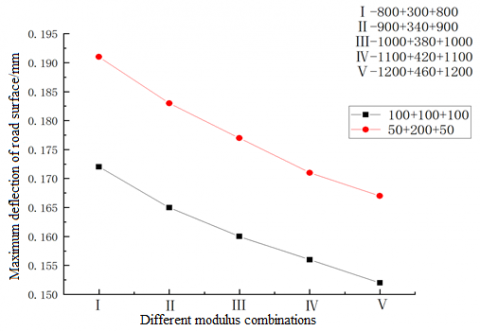Figure 3. Deflection of the pavement under conditions of different modulus combinations and a base layer thickness of 300 mm

## 4.png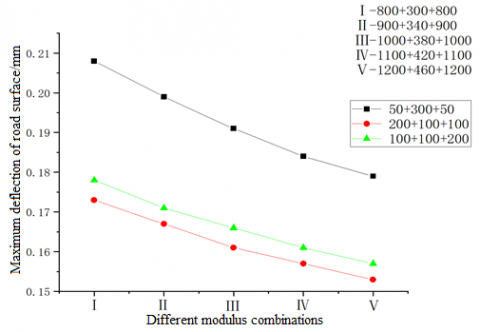Figure 4. Deflection of the pavement under conditions of different modulus combinations and a base layer thickness of 400 mm

## 5.jpg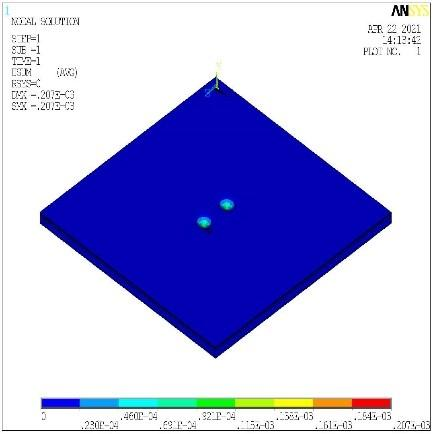Figure 5. Simulation of the deflection of 100+100+100 pavement

Table 3. Deflection of pavements with different structures and modulus values

 Base layer structure Different modulus combinations/Mpa Maximum deflection of road surface/mm 100+100+100 800+300+800 0.172 900+340+900 0.165 1000+380+1000 0.160 1100+420+1100 0.156 1200+460+1200 0.152 50+200+50 800+300+800 0.191 900+340+900 0.183 1000+380+1000 0.177 1100+420+1100 0.171 1200+460+1200 0.167 100+200+100 800+300+800 0.191 900+340+900 0.183 1000+380+1000 0.176 1100+420+1100 0.171 1200+460+1200 0.166 50+300+50 800+300+800 0.208 900+340+900 0.199 1000+380+1000 0.191 1100+420+1100 0.184 1200+460+1200 0.179 200+100+100 800+300+800 0.173 900+340+900 0.167 1000+380+1000 0.161 1100+420+1100 0.157 1200+460+1200 0.153 100+100+200 800+300+800 0.178 900+340+900 0.171 1000+380+1000 0.166 1100+420+1100 0.161 1200+460+1200 0.157

4.2 Tensile stress at the bottom of bottom base layer

## 6.png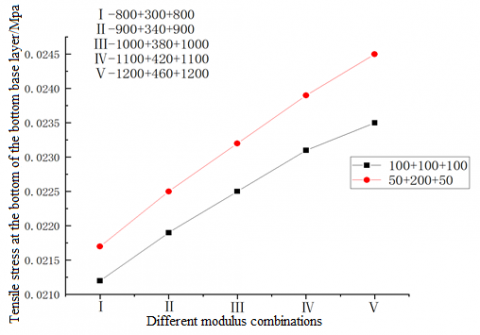Figure 6. Tensile stress at the bottom of bottom base layer under the conditions of different modulus combinations and a base layer thickness of 300 mm

## 7.png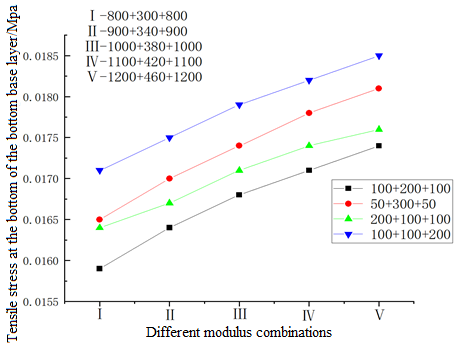Figure 7. Tensile stress at the bottom of bottom base layer under the conditions of different modulus combinations and a base layer thickness of 400 mm

When the tensile stress at the bottom of bottom base layer is greater than the allowable tensile stress at the bottom of the base layer, cracks will appear in the base layer, and to a certain extent, the growth speed the cracks spreading to the surface layer is determined by the thickness of the base layer, namely the structure combination of the base layer (Figure 6, Figure 7). The too-thick base layer will greatly increase the construction cost of the pavement, during construction, it’ll be difficult to compact the pavement, moreover, after putting into real use, the pavement is prone to wheel rutting and other damages, which will seriously affect the quality of the pavement (Figure 8). If the thickness of the base layer is too small, the bottom layer stress is not obvious, therefore, a good choice of base layer structure combination can prevent the generation of cracks. According to the results of finite element analysis shown below, it’s obtained that the base layers of different modulus combinations had also met the specifications of tensile stress at the bottom of base layer in Table 4.

## 8.jpg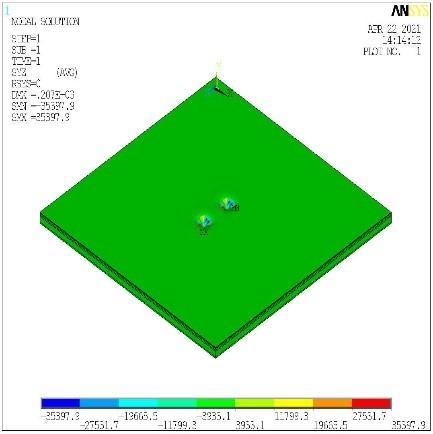Figure 8. Tensile stress at the bottom of bottom base layer of the 100+100+100 pavement

Table 4. Vertical compressive strain on the top surface of roadbed with different base structure types

 Base layer structure Different modulus combinations/Mpa Tensile stress at the bottom of bottom base layer/Mpa 100+100+100 800+300+800 0.021188 900+340+900 0.021894 1000+380+1000 0.022511 1100+420+1100 0.023057 1200+460+1200 0.023545 50+200+50 800+300+800 0.02167 900+340+900 0.025509 1000+380+1000 0.023249 1100+420+1100 0.023908 1200+460+1200 0.024502 100+200+100 800+300+800 0.015931 900+340+900 0.016371 1000+380+1000 0.016755 1100+420+1100 0.017094 1200+460+1200 0.017396 50+300+50 800+300+800 0.016455 900+340+900 0.016957 1000+380+1000 0.017396 1100+420+1100 0.017784 1200+460+1200 0.01813 200+100+100 800+300+800 0.016358 900+340+900 0.016742 1000+380+1000 0.017073 1100+420+1100 0.017363 1200+460+1200 0.017619 100+100+200 800+300+800 0.017111 900+340+900 0.017525 1000+380+1000 0.017882 1100+420+1100 0.018194 1200+460+1200 0.018471

With the help of the constructed model, the tensile stress at the bottom of bottom base layer under different base layer structures and different elastic modulus values were simulated, and the results showed that for each structure type, the tensile stress at the bottom of bottom base layer had all met the specifications. After analyzing the data, it’s concluded that, under a same thickness, the thicker the cement stabilized macadam, the smaller the tensile stress at the bottom of the bottom base layer of the pavement. The thicker the graded crushed stone, the higher the growth rate of tensile stress at the bottom of the bottom base layer. Under the condition of a same type of base layer structure, if the cement stabilized macadam is placed in the bottom base layer, the tensile stress at the bottom of the bottom base layer of the pavement will increase slightly.

4.3 Vertical compressive strain on the top surface of roadbed

## 9.png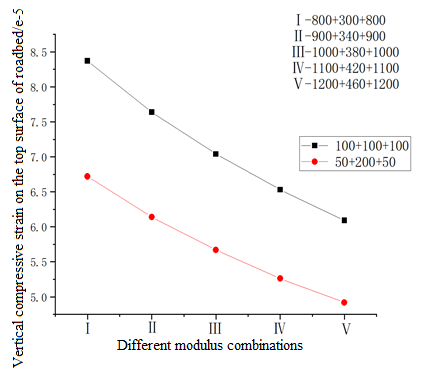Figure 9. Vertical compressive strain on the top surface of roadbed under the conditions of different modulus combinations and a base layer thickness of 300 mm

## 10.pngFigure 10. Vertical compressive strain on the top surface of roadbed under the conditions of different modulus combinations and a base layer thickness of 400 mm

The obtained values showed that, under each structure, the vertical compressive strain on the top surface of roadbed had all met the specifications. After analyzing the data, it’s concluded that, under a same thickness, the thicker the cement stabilized macadam, the smaller the vertical compressive strain on the top surface of roadbed (Figure 9, Figure 10). The thicker the graded broken stone used in the pavement, the lower the growth rate of vertical compressive strain on the top surface of roadbed. Under the condition of a same type of base layer structure (Figure 11), if the cement stabilized macadam is placed in the bottom base layer, the vertical compressive strain on the top surface of roadbed will increase slightly in Table 5.

Table 5. Vertical compressive strain on the top surface of roadbed under different base layer structures

 Base layer structure Different modulus combinations/Mpa Vertical compressive strain on the top surface of roadbed 100+100+100 800+300+800 -8.37e-5 900+340+900 -7.64e-5 1000+380+1000 -7.04e-5 1100+420+1100 -6.53e-5 1200+460+1200 -6.09e-5 50+200+50 800+300+800 -6.72e-5 900+340+900 -6.14e-5 1000+380+1000 -5.67e-5 1100+420+1100 -5.26e-5 1200+460+1200 -4.92e-5 100+200+100 800+300+800 -5.6e-5 900+340+900 -5.11e-5 1000+380+1000 -4.7e-5 1100+420+1100 -4.36e-5 1200+460+1200 -4.07e-5 50+300+50 800+300+800 -4.76e-5 900+340+900 -4.34e-5 1000+380+1000 -4.0e-5 1100+420+1100 -3.71e-5 1200+460+1200 -3.46e-5 200+100+100 800+300+800 -6.02e-5 900+340+900 --5.47e-5 1000+380+1000 -5.02e-5 1100+420+1100 -4.63e-5 1200+460+1200 -4.31e-5 100+100+200 800+300+800 -6.25e-5 900+340+900 -5.69e-5 1000+380+1000 -5.22e-5 1100+420+1100 -4.83e-5 1200+460+1200 -4.49e-5

## 11.jpg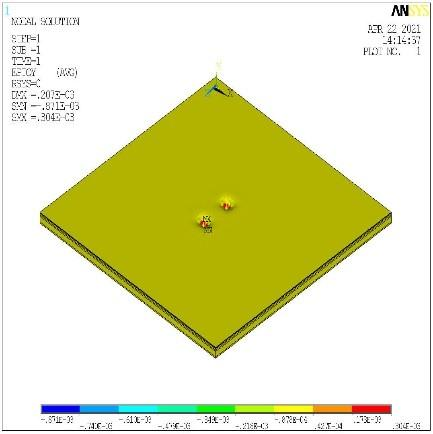Figure 11. Vertical compressive strain on the top surface of roadbed of the 100+100+100 pavement

5. Conclusion

By conducting numerical simulation on base layer structures of different thickness and modulus combinations, this paper obtained a new-type pavement structure, the CGC structure. Simulation data showed that, the proposed structure had met relevant specifications in terms of deflection value, tensile stress at the bottom of bottom base layer, and vertical strain on the top surface of roadbed. In engineering projects in the future, by reasonably controlling the CGC structure and modulus of the pavement, the design ideas of suppressing crack generation and optimizing pavement structure could be realized, which has a certain reference value for the structure design of asphalt pavement.

References

 Assogba, O.C., Sun, Z., Tan, Y., Nonde, L., Bin, Z. (2020). Finite-element simulation of instrumented asphalt pavement response under moving vehicular load. International Journal of Geomechanics, 20(3): 04020006. https://doi.org/10.1061/(ASCE)GM.1943-5622.0001616

 Zhao, B.W. (2020). Mechanical response analysis of semi -rigid base asphalt pavement based on different bonding conditions. Northern Communications, 11: 66-69. https://doi.org/10.15996/j.cnki.bfjt.2020.11.017

 Zhao, J. (2020). Comparative analysis of asphalt pavement structure between semi-rigid base and flexible base. China Housing Facilities, 6: 97, 112.

 Lv, S., Yuan, J., Peng, X., Cabrera, M.B., Liu, H., Luo, X., You, L. (2021). Standardization to evaluate the lasting capacity of rubberized asphalt mixtures with different testing approaches. Construction and Building Materials, 269: 121341. https://doi.org/10.1016/j.conbuildmat.2020.121341

 Zhang, R.Z., Ling, T.Q., Yuan, M., Ning, H.Y. (2011). Influence of Asphalt Semi-rigid Base Modules on Pavement Structural Stress. Journal of Chongqing Jiaotong University (Natural Science), 30(4): 755-758, 863.

 Si, W.B. (2017). Analysis of Asphalt Pavement Structure with Combined Base Course Based on Finite Element. Chongqing Jiaotong University.

 Wang, X., Ma, X. (2020). Responses of semi-rigid base asphalt pavement with interlayer contact bonding model. Advances in Civil Engineering, 2020: 8841139. https://doi.org/10.1155/2020/8841139

 Luo, Y.S. (2016). The influence analysis of structural parameters on the mechanical behavior of asphalt pavement. Shanxi Science & Technology of Communications, 3: 26-28.

 Hu, X.D., Sun, L.J. (2005). Measuring tire ground pressure distribution of heavy vehicle. Journal-Tongji University, 33(11): 1443.

 Dong, H.B. (2012). Indoor Evaluation Test Research on Top-down Cracks of Asphalt Pavement. Changan University.

 Wang, G., Zhang. Y.B. (2020). Finite Element Analysis of Asphalt Pavement Structure with Semi-rigid Base Under Overload. Journal of Wuhan University of Technology(Transportation Science & Engineering), 44(3): 579-583. https://doi.org/10.3963/j.issn.2095-3844.2020.03.035